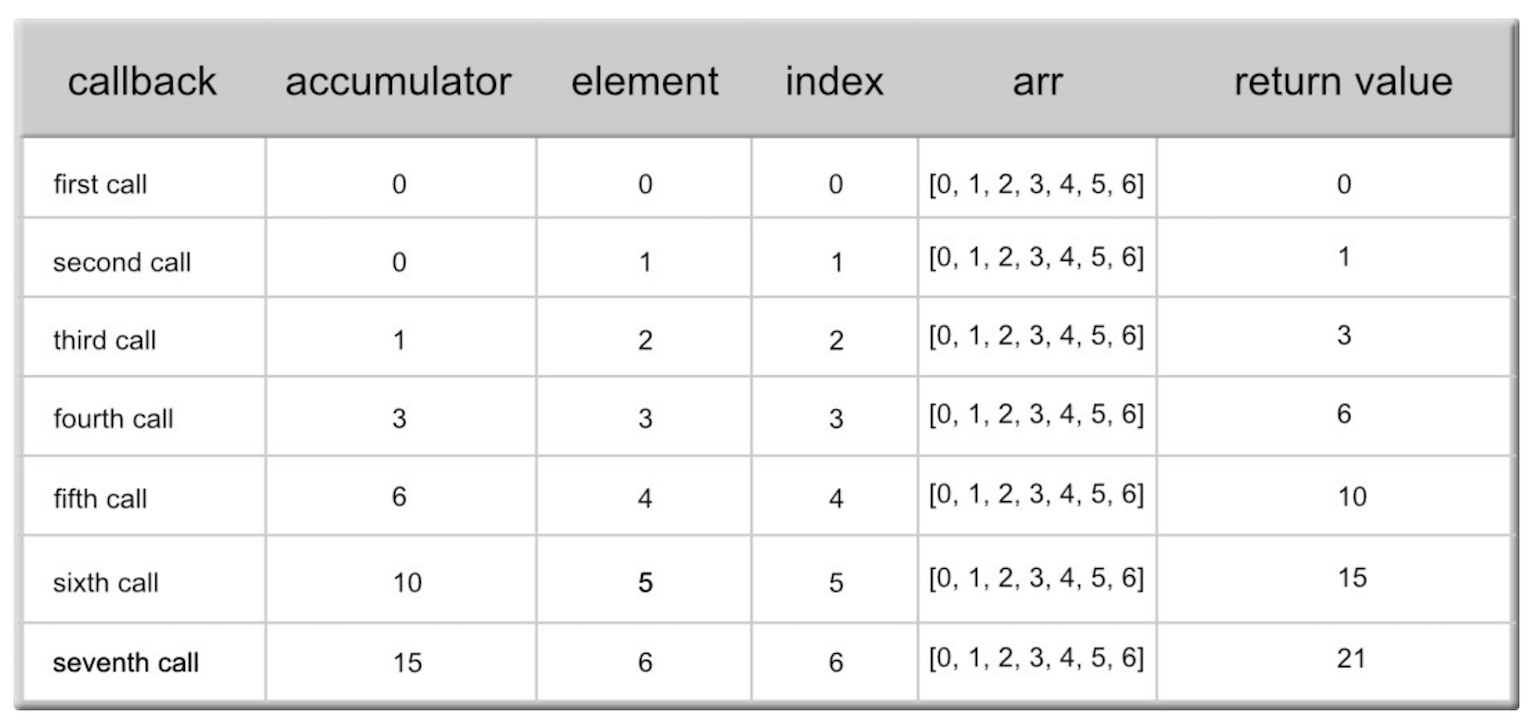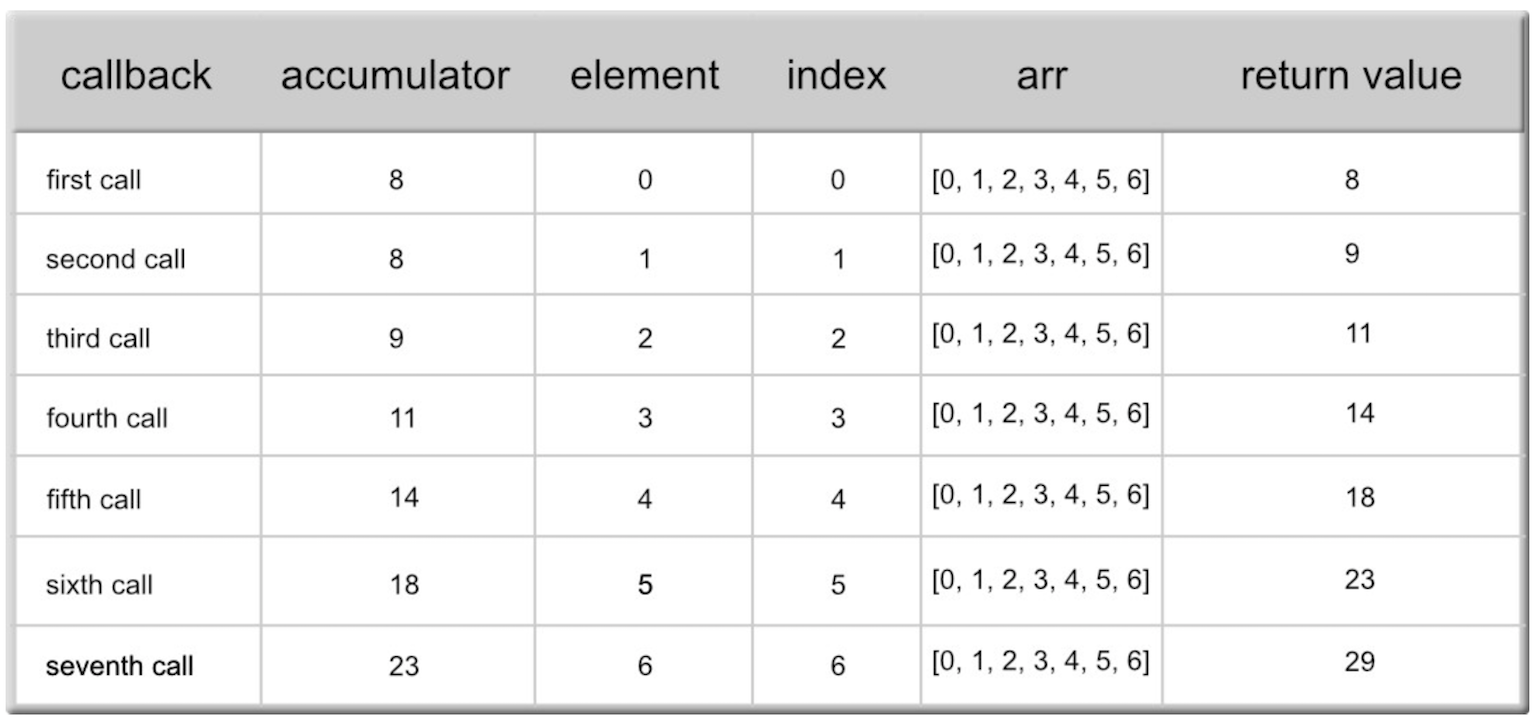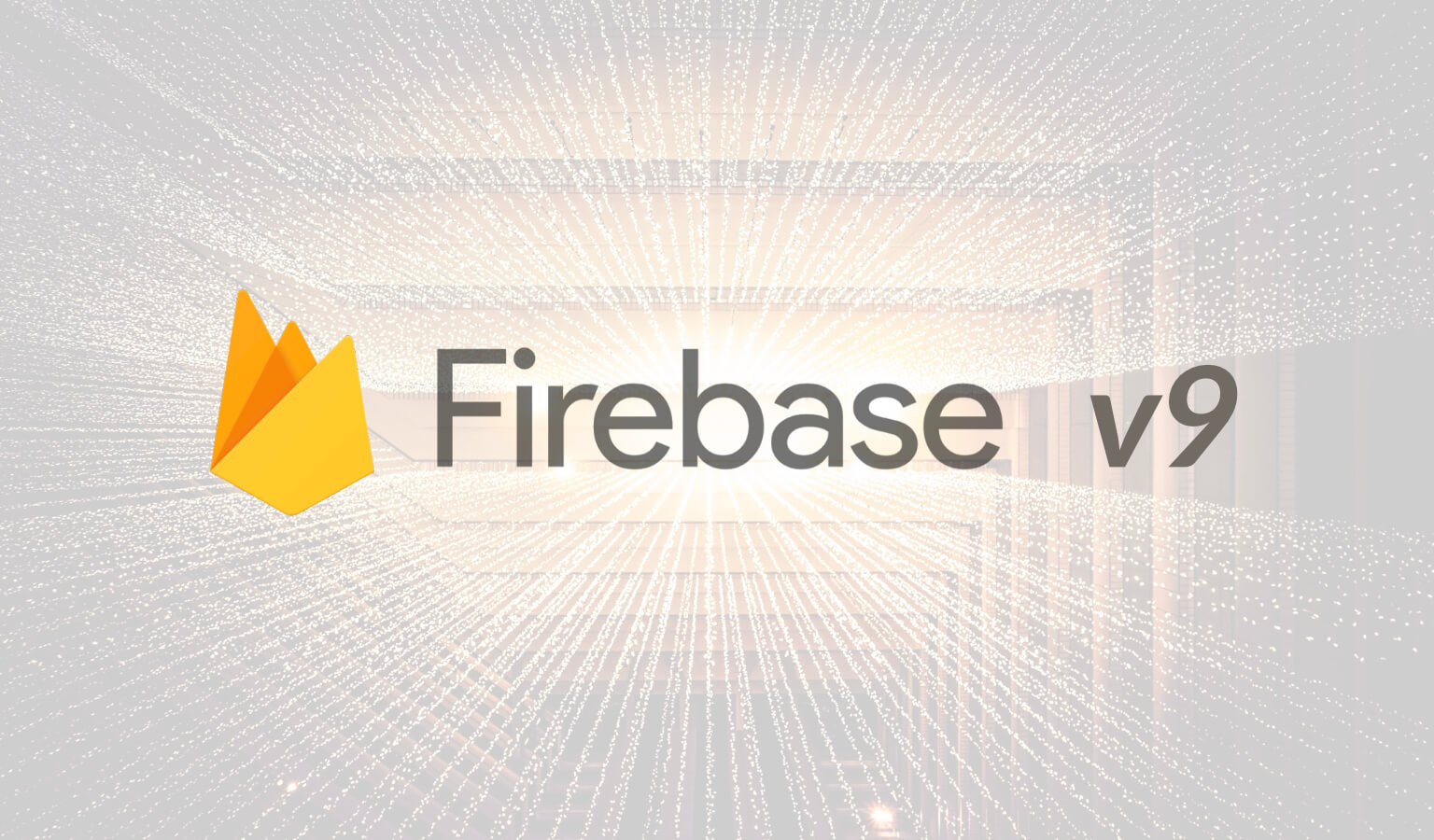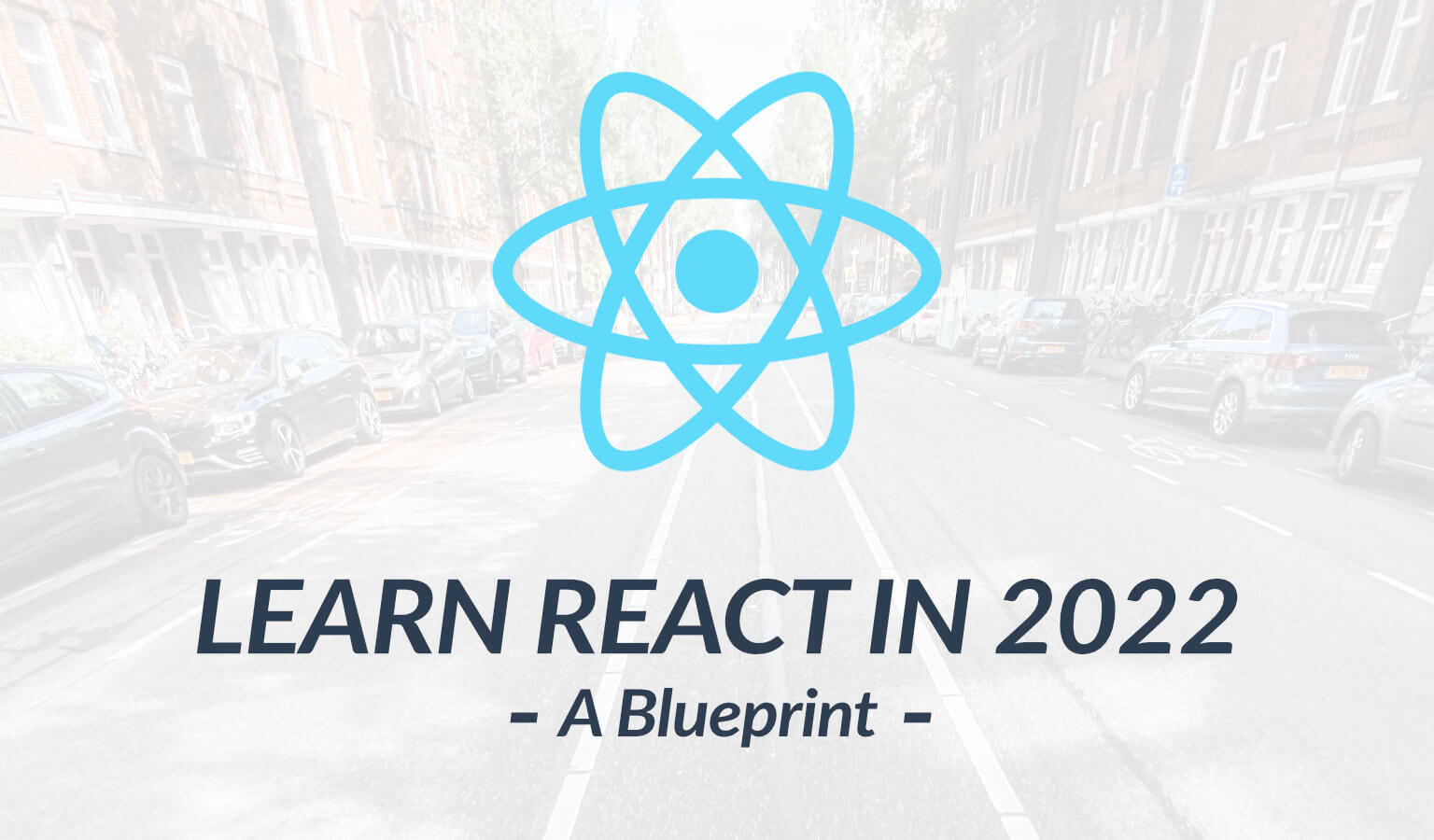# Understanding the JavaScript Reduce Method

One of the hardest JavaScript methods to grasp is the reduce method, yet it is very powerful. In this post, I'll break it down for you step-by-step.

## What is the JavaScript reduce method?

The reduce() method loops through an array, executing a function for each value of the array, from left to right, and reduces the array to a single value.

Breaking it down it…

• loops through an array
• executes a function for each element of the array
• and reduces the array to a single value

To better understand this let's just walk through the syntax step by step. Let's build out an empty function to demonstrate.

Let's say we have an array called arrayExample. Let's first attach our method to it.

``arrayExample.reduce()``

Second, let's enter a callback function. This is the function that will be executed on every element as it loops through an array:

``````arrayExample.reduce(function(){

})``````

Third, let's talk about the reduce method parameters as this is what trips everyone up: The callback function has four parameters:

1. accumulator – accumulates all of the callbacks' returned values (required).
2. element – value of the current element (required)
3. index – index of the current element (optional)
4. arr – the original array object (optional)

The element is simply the array element as it loops through each time. Index is….well the index. Arr is the array object, and I have no clue the value of this array object.

But what is this accumulator?

Well, think about this: If we are calling a function on each element, and these elements will be reduced into one single value, there needs to be a specified value where the accumulation happens, a value that totals up the changes into a single value. This is the accumulator!!

You will often see this called 'total' or 'acc'. Let's use acc in our examples.

So let's tally it up to the final syntax:

``````arrayExample.reduce(function(acc, element, index, arr){

})``````

### Example 1

Let's try out a simple example to start:

Let's take an array, and reduce the elements into one final sum.

Open up the console below and type an array such as:

``var numbers = [0, 1, 2, 3, 4, 5, 6];``

So whenever I work with the reduce method (as well as forEach, filter, and map), I always use this four step method to build out the method:

First, add the method to the end of our array:

``numbers.reduce();``

Second, add the callback function that will be called on each element. (You can also call an already pre-defined function instead of a callback)

``````numbers.reduce(function(){

})``````

Third, we will enter our accumulator (required) and our current element (required) as arguments:

``````numbers.reduce(function(acc, element){

})``````

Finally, we will enter a condition into our function that adds the elements.

``````numbers.reduce(function(acc, element){
return acc + element;
})``````

And we get 21!

Great!

Now here is what it is doing, broken down:### One More Parameter

Now there is ONE MORE parameter and it's called:

initialValue;

So in total we have:

``````arrayExample.reduce(function(acc, element, index, arr){

}, initialValue)``````

And simply put, the initialValue is the value by which the accumulator will start on. Let's try an example using the initialValue:

Let's take a look at the same example as above, but using an initialValue.

### Example 2

So based on our example above, here is our array again:

``var numbers = [0, 1, 2, 3, 4, 5, 6];``

…and here is our reduce function with the initialValue of 8:

``````numbers.reduce(function(acc, element){
return acc + element;
}, 8)``````

Now what this does, is it starts the accumulator at 8, giving us a return value of 29. Here is the breakdown showing the difference. See how the accumulator begins with 8?:*** If there is no initialValue provided, the accumulator starts as the value of the first array element.

If there is an initialValue, this will be the initial value of the accumulator from the start. Okay, not too bad.

### Example 3

Let's look at a final example to assure that we understand the basics of reduce().

Let's look at some data (State and Population):

``````var population = [
{
state: 'California',
pop: 39780000,
},
{
state: 'Virginia',
pop: 8412000,
},
{
state: 'Florida',
pop: 20610000,
},
{
state: 'Maine',
pop: 1331000,
},
]``````

Let's use the reduce() method to get the total population of our four states.

Why don't you give it a shot? Add up the populations (using element.pop) using reduce().

Ok, so let's follow the 4-step process again:

First, we'll attach the reduce() method:

``population.reduce()``

Second, our callback function:

``````population.reduce(function(){

})``````

Third, our arguments (acc and element are required):

``````population.reduce(function(acc, element){

})``````

Fourth, our conditions:

``````population.reduce(function(acc, element){
return element.pop + acc;
})``````

Now what did you get?

Did you get 1331000206100008412000?

If you did, you are wrong, BUT this is a great thing because the lesson will much more beneficial than the mistake.

You see, if you did not set an initial value, then you actually started off with the number 39780000 initially!!!

Instead, we need to start off with an initialValue of 0. So try it with the 0.

``````population.reduce(function(acc, element){

return element.pop + acc;

}, 0)``````

..and you see that we now get the correct answer of 70133000.

Great work in tackling an initially difficult, but extremely powerful, method.

----------

About MeTravis of

#### Travis Media

Who Am I? I was 34 years old in a job I hated when I decided to learn to code. Read More
Recent Posts
Explore

### You May Also Like### Navigating S3 Using the AWS SDK for Javascript: A Guide

In this post, we'll look at how to navigate S3 using the AWS SDK for …### How to use Firebase v9 with React (setup, authentication and CRUD examples)

Firebase v9 takes a modular approach which is different from previous …### 5 Steps for Learning React JS in 2022: A Blueprint

Planning on learning React JS in 2022? This React JS learning roadmap …